# Instrument Response Functions (DL3)#

Typically the IRFs are stored in the form of multidimensional tables giving the response functions such as the distribution of gamma-like events or the probability density functions of the reconstructed energy and position.

## Expected number of detected events#

To model the expected number of events a gamma-ray source should produce on a detector one has to model its effect using an instrument response function (IRF). In general, such a function gives the probability to detect a photon emitted from true position $$p_{\rm true}$$ on the sky and true energy $$E_{\rm true}$$ at reconstructed position $$p$$ and energy $$E$$ and the effective collection area of the detector at position $$p_{\rm true}$$ on the sky and true energy $$E_{\rm true}$$.

We can write the expected number of detected events $$N(p, E)$$:

$N(p, E) {\rm d}p {\rm d}E = t_{\rm obs} \int_{E_{\rm true}} {\rm d}E_{\rm true} \, \int_{p_{\rm true}} {\rm d}p_{\rm true} \, R(p, E|p_{\rm true}, E_{\rm true}) \times \Phi(p_{\rm true}, E_{\rm true})$

where:

• $$R(p, E| p_{\rm true}, E_{\rm true})$$ is the instrument response (unit: $${\rm m}^2\,{\rm TeV}^{-1}$$)

• $$\Phi(p_{\rm true}, E_{\rm true})$$ is the sky flux model (unit: $${\rm m}^{-2}\,{\rm s}^{-1}\,{\rm TeV}^{-1}\,{\rm sr}^{-1}$$)

• $$t_{\rm obs}$$ is the observation time: (unit: $${\rm s}$$)

## Factorisation of the IRFs#

Most of the time, in high level gamma-ray data (DL3), we assume that the instrument response can be simplified as the product of three independent functions:

$R(p, E|p_{\rm true}, E_{\rm true}) = A_{\rm eff}(p_{\rm true}, E_{\rm true}) \times PSF(p|p_{\rm true}, E_{\rm true}) \times E_{\rm disp}(E|p_{\rm true}, E_{\rm true}),$

where:

• $$A_{\rm eff}(p_{\rm true}, E_{\rm true})$$ is the effective collection area of the detector (unit: $${\rm m}^2$$). It is the product of the detector collection area times its detection efficiency at true energy $$E_{\rm true}$$ and position $$p_{\rm true}$$.

• $$PSF(p|p_{\rm true}, E_{\rm true})$$ is the point spread function (unit: $${\rm sr}^{-1}$$). It gives the probability of measuring a direction $$p$$ when the true direction is $$p_{\rm true}$$ and the true energy is $$E_{\rm true}$$. Gamma-ray instruments consider the probability density of the angular separation between true and reconstructed directions $$\delta p = p_{\rm true} - p$$, i.e. $$PSF(\delta p|p_{\rm true}, E_{\rm true})$$.

• $$E_{\rm disp}(E|p_{\rm true}, E_{\rm true})$$ is the energy dispersion (unit: $${\rm TeV}^{-1}$$). It gives the probability to reconstruct the photon at energy $$E$$ when the true energy is $$E_{\rm true}$$ and the true position $$p_{\rm true}$$. Gamma-ray instruments consider the probability density of the migration $$\mu=\frac{E}{E_{\rm true}}$$, i.e. $$E_{\rm disp}(\mu|p_{\rm true}, E_{\rm true})$$.

The implicit assumption here is that energy dispersion and PSF are completely independent. This is not totally valid in some situations.

These functions are obtained through Monte-Carlo simulations of gamma-ray showers for different observing conditions, e.g. detector configuration, zenith angle of the pointing position, detector state and different event reconstruction and selection schemes. In the DL3 format, the IRF are distributed for each observing run.

Further details on individuals responses and how they are implemented in gammapy are given in Effective area, Energy Dispersion and Point Spread Function.

Most of the formats defined at IRFs are supported. At the moment, there is little support for Fermi-LAT or other instruments.

Most users will not use gammapy.irf directly, but will instead use IRFs as part of their spectrum, image or cube analysis to compute exposure and effective EDISP and PSF for a given dataset.

## IRF axis naming#

In the IRF classes we use the following axis naming convention:

Variable

Definition

energy

Reconstructed energy axis ($$E$$)

energy_true

True energy axis ($$E_{\rm true}$$)

offset

Field of view offset from center ($$p_{\rm true}$$)

fov_lon

Field of view longitude

fov_lat

Field of view latitude

migra

Energy migration ($$\mu$$)

rad

Offset angle from source position ($$\delta p$$)

## Using gammapy.irf#

### Examples using gammapy.irf.PSFMap#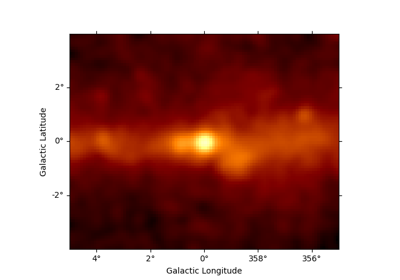Fermi-LAT with Gammapy

Fermi-LAT with Gammapy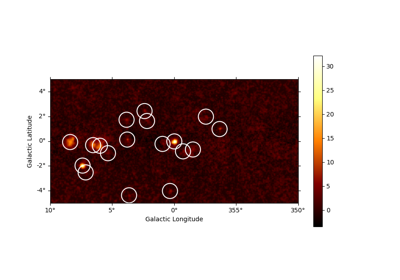Source detection and significance maps

Source detection and significance maps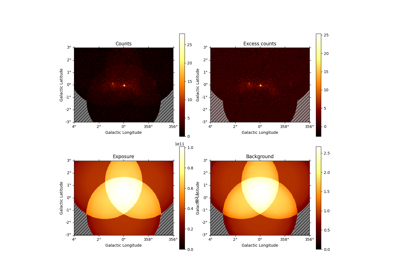Datasets - Reduced data, IRFs, models

Datasets - Reduced data, IRFs, models

### Examples using gammapy.irf.EDispKernelMap#Fermi-LAT with Gammapy

Fermi-LAT with GammapySource detection and significance maps

Source detection and significance maps

### Examples using gammapy.irf.load_cta_irfs#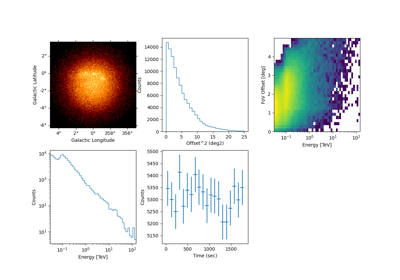CTA with Gammapy

CTA with Gammapy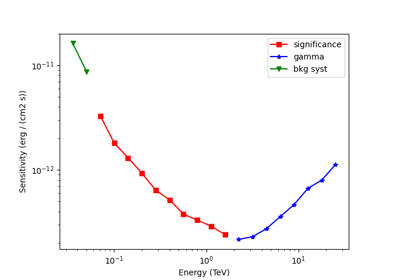Point source sensitivity

Point source sensitivity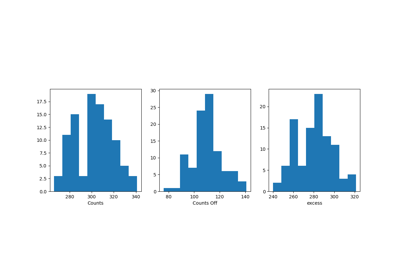1D spectrum simulation

1D spectrum simulation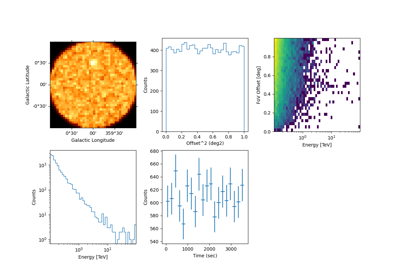Event sampling

Event sampling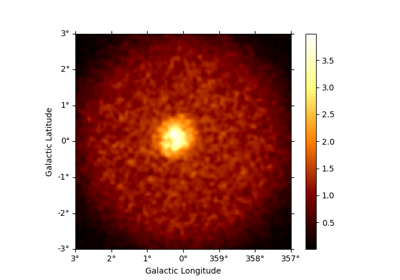3D map simulation

3D map simulation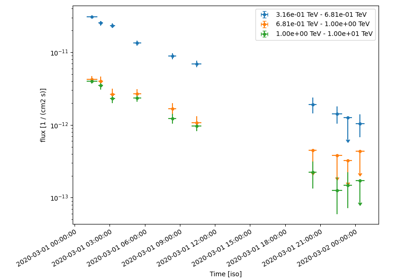Simulating and fitting a time varying source

Simulating and fitting a time varying source